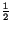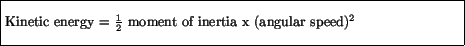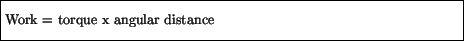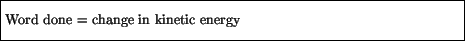Next: Angular momentum Up: Rotational Motion Previous: Moment of inertia

# Rotational kinetic energy

Recall that an object of a certain mass moving with particular speed will have an associated kinetic energymass x speed2. An object spinning about an axis will also have associated with it a kinetic energy, composed of the kinetic energies of each individual part of the object. These individual contributions may be summed up to give an expression for the total kinetic energy of the spinning object:As with linear motion, where a force did work on an object and led to a change in the object's kinetic energy, for rotational motion the work done by a torque:goes into changing the rotational kinetic energy of an object:The rotational kinetic energy is treated like any other form of energy, in that it can be transformed into other forms (eg, potential), and also it is a component of the (conserved) total energy of a system.

Sometimes objects rotate about an axis that is itself in motion. For example, if you roll a cylinder down a ramp without any slipping, the axis about which it rotates (the center of the cylinder) moves down the ramp. In this case the total kinetic energy of the cylinder is the sum of its rotational kinetic energy plus its translational kinetic energy. It is therefore easy to see that an object with a higher moment of inertia will take longer to roll down the ramp. As a specific case consider a solid cylinder and a hollow cylinder with the same mass and radius starting at rest and rolling down the same ramp side by side. Which do you expect will reach the bottom first? We know that the moment of inertia of the hollow cylinder is greater, so that for a given angular velocity it has more rotational kinetic energy. Moreover, if they both roll without slipping the linear velocity (and hence linear kinetic energy) is the same for both cylinders when their angular velocity is the same. In addition, the change in gravitational potential energy is the same for both cylinders (the weight of the cylinder times the height of the ramp), so both cylinders must have the same total kinetic energy at the bottom of the ramp. This necessarily implies that the angular velocity (and linear velocity) of the hollow cylinder must be less at the bottom of the ramp than that of solid cylinder (otherwise it would have more kinetic energy). Consequently we can conclude that the hollow cylinder moves slower and the solid cylinder arrives at the bottom first.Next: Angular momentum Up: Rotational Motion Previous: Moment of inertia
modtech@theory.uwinnipeg.ca
1999-09-29